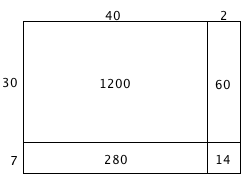### Home > MC1 > Chapter 3 > Lesson 3.3.1 > Problem3-63

3-63.

Draw a generic rectangle to multiply $37·42$.1. Write an addition sentence to show the total area of the generic rectangle.

What are the smaller areas which combine to make the total area of the rectangle?

$1200+280+60+14=1554$

2. Multiply $37·42$ by the standard algorithm, that is, by placing one of the numbers under the other and using the procedure you were taught in previous courses. Show your work. Compare your result with your answer from part (a).

You should remember this method from previous math classes. To get you started, here is the correct set-up:
$\;\;\;42\\\underline{\times37\\}\;$

When you complete this problem, you should have the same answer as in part (a).

3. What method do you prefer? Why?